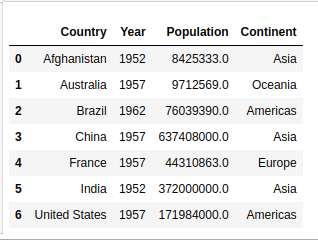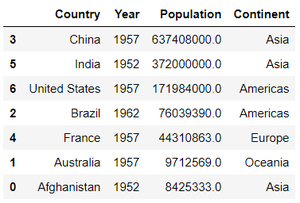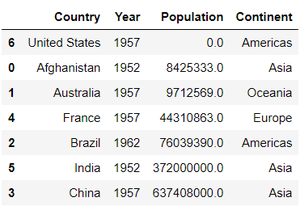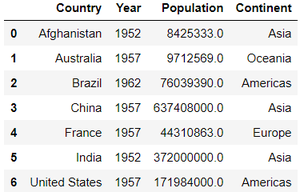Skip to content
Related Articles
How to Sort Pandas DataFrame?
• Last Updated : 28 Jul, 2020

In this article, we will discuss how to sort Pandas Dataframe. Let’s create a dataframe.

Example :

## Python3

 `# importing pandas library``import` `pandas as pd`` ` `# creating and initializing a nested list``age_list ``=` `[[``'Afghanistan'``, ``1952``, ``8425333``, ``'Asia'``],``            ``[``'Australia'``, ``1957``, ``9712569``, ``'Oceania'``],``            ``[``'Brazil'``, ``1962``, ``76039390``, ``'Americas'``],``            ``[``'China'``, ``1957``, ``637408000``, ``'Asia'``],``            ``[``'France'``, ``1957``, ``44310863``, ``'Europe'``],``            ``[``'India'``, ``1952``, ``3.72e``+``08``, ``'Asia'``],``            ``[``'United States'``, ``1957``, ``171984000``, ``'Americas'``]]`` ` `# creating a pandas dataframe``df ``=` `pd.DataFrame(age_list, columns``=``[``'Country'``, ``'Year'``,``                                     ``'Population'``, ``'Continent'``])`` ` `df`

Output :## Sorting Pandas Data Frame

In order to sort the data frame in pandas, function sort_values() is used. Pandas sort_values() can sort the data frame in Ascending or Descending order.

Example 1: Sorting the Data frame in Ascending order

## Python3

 `# importing pandas library``import` `pandas as pd`` ` `# creating and initializing a nested list ``age_list ``=` `[[``'Afghanistan'``,``1952``,``8425333``,``'Asia'``],``            ``[``'Australia'``,``1957``,``9712569``,``'Oceania'``],``            ``[``'Brazil'``,``1962``,``76039390``,``'Americas'``],``            ``[``'China'``,``1957``,``637408000``,``'Asia'``],``            ``[``'France'``,``1957``,``44310863``,``'Europe'``],``            ``[``'India'``,``1952``,``3.72e``+``08``,``'Asia'``],``            ``[``'United States'``,``1957``,``171984000``,``'Americas'``]]`` ` `# creating a pandas dataframe``df ``=` `pd.DataFrame(age_list,columns``=``[``'Country'``,``'Year'``,``                                    ``'Population'``,``'Continent'``])`` ` `# Sorting by column 'Country'``df.sort_values(by``=``[``'Country'``])`

Output :Example 2: Sorting the Data frame in Descending order

## Python3

 `# Sorting Pandas Dataframe in Descending Order`` ` `# importing pandas library``import` `pandas as pd`` ` `# Initializing the nested list with Data set``age_list ``=` `[[``'Afghanistan'``, ``1952``, ``8425333``, ``'Asia'``],``            ``[``'Australia'``, ``1957``, ``9712569``, ``'Oceania'``],``            ``[``'Brazil'``, ``1962``, ``76039390``, ``'Americas'``],``            ``[``'China'``, ``1957``, ``637408000``, ``'Asia'``],``            ``[``'France'``, ``1957``, ``44310863``, ``'Europe'``],``            ``[``'India'``, ``1952``, ``3.72e``+``08``, ``'Asia'``],``            ``[``'United States'``, ``1957``, ``171984000``, ``'Americas'``]]`` ` `# creating a pandas dataframe``df ``=` `pd.DataFrame(age_list, columns``=``[``'Country'``, ``'Year'``,``                                     ``'Population'``, ``'Continent'``])`` ` `# Sorting by column "Population"``df.sort_values(by``=``[``'Population'``], ascending``=``False``)`

Output :Example 3: Sorting Pandas Data frame by putting missing values first

## Python3

 `# Sorting Pandas Data frame by putting``# missing values first`` ` `# importing pandas library``import` `pandas as pd`` ` `# Initializing the nested list with Data set``age_list ``=` `[[``'Afghanistan'``, ``1952``, ``8425333``, ``'Asia'``],``            ``[``'Australia'``, ``1957``, ``9712569``, ``'Oceania'``],``            ``[``'Brazil'``, ``1962``, ``76039390``, ``'Americas'``],``            ``[``'China'``, ``1957``, ``637408000``, ``'Asia'``],``            ``[``'France'``, ``1957``, ``44310863``, ``'Europe'``],``            ``[``'India'``, ``1952``, ``3.72e``+``08``, ``'Asia'``],``            ``[``'United States'``, ``1957``, ``0``, ``'Americas'``]]`` ` `# creating a pandas dataframe``df ``=` `pd.DataFrame(age_list, columns``=``[``'Country'``, ``'Year'``,``                                     ``'Population'``, ``'Continent'``])`` ` `# Sorting by column "Population"``# by putting missing values first``df.sort_values(by``=``[``'Population'``], na_position``=``'first'``)`

Output :Example 4:  Sorting Data frames by multiple columns

## Python3

 `#  Sorting Pandas Dataframe based on``# the Values of Multiple Columns`` ` `# importing pandas library``import` `pandas as pd`` ` `# Initializing the nested list with data set``age_list ``=` `[[``'Afghanistan'``, ``1952``, ``8425333``, ``'Asia'``],``            ``[``'Australia'``, ``1957``, ``9712569``, ``'Oceania'``],``            ``[``'Brazil'``, ``1962``, ``76039390``, ``'Americas'``],``            ``[``'China'``, ``1957``, ``637408000``, ``'Asia'``],``            ``[``'France'``, ``1957``, ``44310863``, ``'Europe'``],``            ``[``'India'``, ``1952``, ``3.72e``+``08``, ``'Asia'``],``            ``[``'United States'``, ``1957``, ``171984000``, ``'Americas'``]]`` ` `# creating a pandas dataframe``df ``=` `pd.DataFrame(age_list, columns``=``[``'Country'``, ``'Year'``,``                                     ``'Population'``, ``'Continent'``])`` ` ` ` `# Sorting by columns "Country" and then "Continent"``df.sort_values(by``=``[``'Country'``, ``'Continent'``])`

Output :Attention geek! Strengthen your foundations with the Python Programming Foundation Course and learn the basics.

To begin with, your interview preparations Enhance your Data Structures concepts with the Python DS Course. And to begin with your Machine Learning Journey, join the Machine Learning – Basic Level Course

My Personal Notes arrow_drop_up